# Put Option as a Future Sensitivity of Option Prices Assignment Sample

Introduction

The Black Scholes model considers the put option and put option as a function of the future price. This is irrespective of any stock price; it considered the market stock price and a common and constant stock price profits. This helps to explain how an arbitrage profit may be earned in futures markets.The Put option as a Function of the Stock Price Profits

It can be considered that the strike price, the risk rate of interest and the time of expiration of the put option as a derivation for the return of the future stock (Cao, Yu and Zhong, 2010).  Of the five variable that are considered as vital in determination of the Black Scholes model price for the needed put option, the only variable from the analysis that is not observable is shown to be the return on the stock that primarily determines the put option.

Graph 1: Put price as a function of the stock price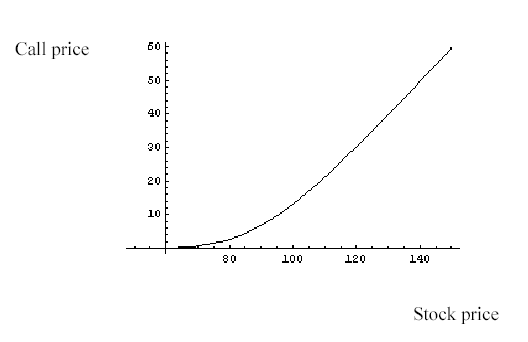From the graph it is evident that a put option gives the investor to opportunity to be able to purchase a stock at a fixed price on as well as before the future date. For example, the investor has a chance to purchase a put option given the right to purchase a share of the company as a price determined by the Model equation of \$80 at a time during the 38 days prior to the due date. Since the investors are never forced to undertake a put option, therefore, the loss considered is limited to the original cost of the put option purchased; futurely the company according to the grapy above is trading well at \$50, the cost of a cell option with more than 38 months to the data of expiration might even cost less than \$10 per share. For example, if the investor is at \$60 in the 38 months he/she will let the put option and the loss us reduced to the value of the purchase of the put option (Cremers and Weinbaum, 2010). However. In case the company is at more than \$100 the investor will have been able to make \$40 per share. This he would have exercised the put option to the stock price and took the stock at \$60 and the selling price \$100.   Thus, an estimate of volatility is conditional from the price of the put option at \$60, (implied volatility). According to the analysis, it is evident that the computation of the estimate is the same in many measure to that of the yield to maturity for a bond. Black Scholes is a more complicated model that is able to depict the values of stock among others in order to offer support in price as well as stock on put option.

Graph 2: Performance of the delta hedge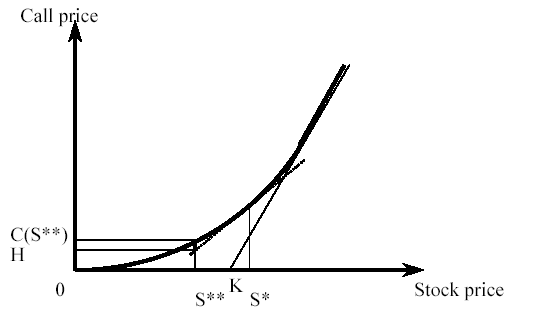Because the delta of a put option depends on the stock price and time, as well as other factors, the position of the price is only short-term of time delta neutral. In practice, it is considered that the stock price portfolio should be balanced so that it can adapt to the changing market. Strategies are needed to manage the stock price risks which considers frequent rebalances denoted as dynamic hedging. It can be pointed out from the Black Scholes equation can be obtained from the analysis of delta hedge whose position is considered a constant delta neutral. However, it is considered that this approach is analogous to the reproduction of a put option to stock price.The Vega of a Put as a function of the Stock Price

From the analysis, the Vega of a put option shows how much the price of an option is able to change as the volatility of the determining asset changes. The Vega used is quoted to indicate the theoretical stock price change for every 1% level in volatility. In this case, the theoretical price was \$2.5 while the Vega was showing \$0.25, this means that the volatility shifts from its normal value of 30% to 33%; again the theoretical stock price will also increase to \$2.80. Therefore, it can be considered that Vega is more sensitive at put option as indicated in the graph below: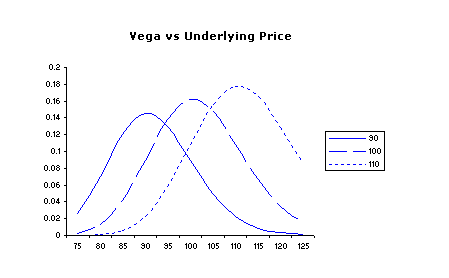Put delta as a function of Stock Price

The below graph shows that the put option has a negative delta, that has its values ranging from -1 to 0. This means that the underlying market price will increase. Likewise, the put option will decrease by the delta value.  In addition, the graph shows that the delta of a put option is able to alter the underlying stock price changes.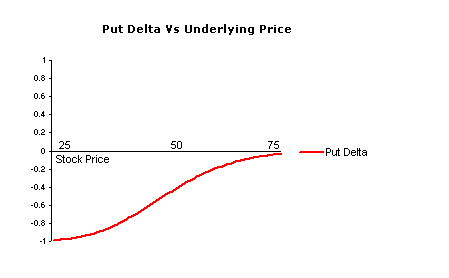Put option as a function of Stock Price

From the analysis it is considered that option is the simplest way to trade stock price. It shows that the price of the asset must shift below the strike stock price of the normal put option before the maturity date (38days) for the option to be profitable.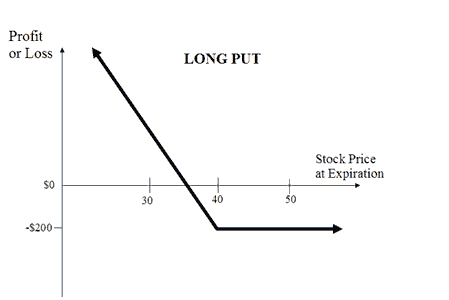Put Vega as a function of Stock Price

The more time the stock has to the maturity period considered in this case as 38 days, the higher the Vega. The graph below shows that the traits of the Vega of put option expire in 20 days when the stock is still trading at \$50.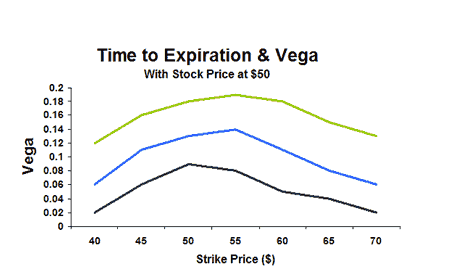Conclusion

It can be concluded that the model has proved to have good characteristics that supports pricing performance but the model has not yet exploited in financial literature since its inception. However, consideration of put option is reasonable based on the underlying model as the best investment option and determination of future profits.